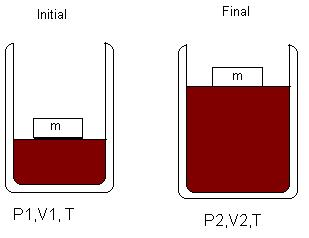# 8.2: Gas Expansion

$$\newcommand{\vecs}{\overset { \rightharpoonup} {\mathbf{#1}} }$$ $$\newcommand{\vecd}{\overset{-\!-\!\rightharpoonup}{\vphantom{a}\smash {#1}}}$$$$\newcommand{\id}{\mathrm{id}}$$ $$\newcommand{\Span}{\mathrm{span}}$$ $$\newcommand{\kernel}{\mathrm{null}\,}$$ $$\newcommand{\range}{\mathrm{range}\,}$$ $$\newcommand{\RealPart}{\mathrm{Re}}$$ $$\newcommand{\ImaginaryPart}{\mathrm{Im}}$$ $$\newcommand{\Argument}{\mathrm{Arg}}$$ $$\newcommand{\norm}{\| #1 \|}$$ $$\newcommand{\inner}{\langle #1, #2 \rangle}$$ $$\newcommand{\Span}{\mathrm{span}}$$ $$\newcommand{\id}{\mathrm{id}}$$ $$\newcommand{\Span}{\mathrm{span}}$$ $$\newcommand{\kernel}{\mathrm{null}\,}$$ $$\newcommand{\range}{\mathrm{range}\,}$$ $$\newcommand{\RealPart}{\mathrm{Re}}$$ $$\newcommand{\ImaginaryPart}{\mathrm{Im}}$$ $$\newcommand{\Argument}{\mathrm{Arg}}$$ $$\newcommand{\norm}{\| #1 \|}$$ $$\newcommand{\inner}{\langle #1, #2 \rangle}$$ $$\newcommand{\Span}{\mathrm{span}}$$

In Gas Expansion, we assume Ideal behavior for the two types of expansions:

## Isothermal ExpansionThis shows the expansion of gas at constant temperature against weight of an object's mass (m) on the piston. Temperature is held constant, therefore the change in energy is zero (U=0). So, the heat absorbed by the gas equals the work done by the ideal gas on its surroundings. Enthalpy change is also equal to zero because the change in energy zero and the pressure and volume is constant.

### Isothermal Irreversible/Reversible processThe graphs clearly show work done (area under the curve) is greater in a reversible process.

Adiabatic means when no heat exchange occurs during expansion between system and surrounding and the temperature is no longer held constant.

This equation shows the relationship between PV and is useful only when it applies to ideal gas and reversible adiabatic change. The equation is very similar to Boyle's law except it has exponent (gamma) due to change in temperature. The work done by an adiabatic reversible process is given by the following equation:

where T2 is less than T1. The internal energy of the system decreases as the gas expands. The work can be calculated in two ways because the Internal energy (U) does not depend on path. The graph shows that less work is done in an adiabatic reversible process than an Isothermal reversible process.## References

1. Chang, Raymond. Physical Chemistry for the Biosciences. Sausalito, CA: University Science, 2005.

8.2: Gas Expansion is shared under a not declared license and was authored, remixed, and/or curated by LibreTexts.